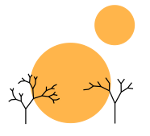# The Continuum Hypothesis Part 2 (The Constructible Universe)

Rimu Shuang. "Untitled Photo". Jan 1, 2014. Under a Creative Commons 3.0 Attribution License.

### continuum_hypothesis, math

This post is going to be tackling the notion of the constructible (or definable) universe L and the axiom V = L, which intuitively states that all sets are “definable”, i.e. there is a first-order way of uniquely stipulating what each set is. The counter-axiom, V ⊋ L, states that some sets are not definable, that is there are some sets which may exist, but which we can never uniquely identify using first-order logic.

Thus, in a certain sense, the axiom V = L is a statement that all sets are “well-behaved” and can be known, rather than remain forever unknown. To begin, a subset of a model M is definable in M if there is a first-order statement ϕ and a tuple of elements from the model $(a_1, a_2, \ldots, a_n) = \overline{a}$ such that $\phi(x, \overline{a})$ holds if and only if x is in the subset. We say that an element x of a model is definable if the subset {x} is definable. Finally, given some set X, the set of definable sets is defined (haha) as DefX = {YXY is definable in X}

The constructible universe L is then constructed in stages Lα and is indexed by the ordinals where

Lα + 1 = DefLα

and in the case of limit ordinals, we have

Lλ = ⋃α < λLα

. Finally, L0 = ∅.

Then the axiom V = L states that xα(x ∈ Lα).

It will turn out to be the case that Lκ for any limit ordinal κ models V = L. I will quickly skim over why such a Lκ satisfies ZF.

The fact that it satisfies the Emptyset axiom is immediate as is the fact that it satisfies Extensionality. It satisfies Pairing due to the fact that if ψ and ϕ each define separate sets in x ∈ Lα and y ∈ Lβ where α, β < κ, the pair of those two sets can be defined via φ where

φ(x) := ∀y ∈ x(ψ(y) ∨ ϕ(y))

and hence {x,y} ∈ Lmax (α, β) + 1.

To see that Lκ satisfies Union, take an arbitrary set S. Let ϕ define the set S. Then ψ(x) := ϕ(⋃x) defines S (note that this works because a = ⋃x is itself shorthand for z(z ∈ a ↔︎ ∃y(z ∈ y ∈ x))).

Because Lκ is a subset of V, it must also obey Foundation. Because ω ∈ Lκ, it must obey Infinity.

Now Powerset and Replacement are slightly more involved to show and so I will save them for the next post (in addition to actually showing V = L)

For those people who might wonder why I’ve used ZF instead of ZFC, it turns out that we get Choice if we assume that V = L, which I will explain in a later post as well, so I will not go into showing Choice here.

Now assuming we’ve shown that Lκ ⊨ ZF, if we could show that Lκ ⊨ V = L, then we would have shown that V = L is consistent with ZF. This (Lκ ⊨ ZF) may seem immediately apparent, but there is a little bit of subtlety here. In particular, if it were the case that LαLκ = Lα we would be done immediately. However, this is not necessarily the case; what happens if Lκ leaves out some of the elements that would otherwise be in Lα? I will save this final step for showing that V = L is consistent with ZF in the next post as well.